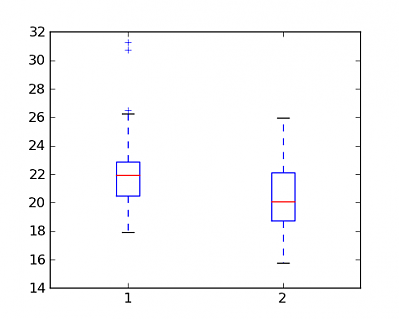# kleemans.ch

## Boxplots

February 11, 2015

I took a statistics course last fall, and there is a lot of code involved for the whole calculation of different parameters.

"Unfortunately" all of this is in R - which is undisputedly one of the best tools for statistics in general - but I don't know it well enough for some good results in a few minutes. So I started using Python with matplotlib.

One example are Boxplots, great for an overview of 5 important parameters: the median, the min and max (if in range), and the 50%-box (IQR).

## Data

The data is from our professor, he provided weight and height of students from some years ago, 250 students in total.

After calculating the BMI entering the data, a first boxplot which can be automatically generated with boxplot(data) looks as follows:So, next up is some fine-tuning to make it look better.

## Axes

At first, I wanted to take out some black lines from the axes, to make it more focussed on the boxplots themselves. I took some good propositions from here.

At first I removed these unnecessary "spines":

``````ax.spines['top'].set_visible(False)
ax.spines['right'].set_visible(False)
ax.spines['bottom'].set_visible(False)``````

Then the ticks:

``````ax.xaxis.set_ticks_position('none')
ax.yaxis.set_ticks_position('none')``````

Then, for better reading, I added some horizintal lines, part of the background grid:

``ax.yaxis.grid(True, linestyle='-', which='major', color='lightgrey', alpha=0.5)``

## Text

For better readability at first sight, some more explanation in the title and on the axes:

``````ax.set_title('BMI-Vergleich von Studierenden')
ax.set_xlabel('Geschlecht')
ax.set_ylabel('BMI')
pylab.xticks([1, 2], ['m', 'w'])``````

## Color

But also the color wasn't what I had on my mind, the blue is quite aggressive. So I added a inidgo tone to all the elements except for the median. This can be done with setting the parameters for each class separately:

``````blue = '#0D4F8B' #indigo
pylab.plt.setp(bp['boxes'], color=blue)
pylab.plt.setp(bp['medians'], color='red')
pylab.plt.setp(bp['whiskers'], color=blue)
pylab.plt.setp(bp['fliers'], color=blue)
pylab.plt.setp(bp['caps'], color=blue)``````

Also, so the picture which is shown (beside the one that is saved) isn't presented in some grey box, you can add `facecolor="white"` when initiating.

So here's the final Boxplot:Python Code (Python 2.7, matplotlib required)

``````'''Plots some boxplots about student BMI data.'''
__date__ = '09.12.2014'

import pylab

# BMI data StatWiSo2003 (m, f)
data = [[17.9163, ... ]]

# create a figure instance
fig = pylab.plt.figure(1, figsize=(9, 6), facecolor="white")
bp = ax.boxplot(data)

# remove axes and ticks
ax.spines['top'].set_visible(False)
ax.spines['right'].set_visible(False)
ax.spines['bottom'].set_visible(False)
ax.xaxis.set_ticks_position('none')
ax.yaxis.set_ticks_position('none')

# some helping lines
ax.yaxis.grid(True, linestyle='-', which='major',
color='lightgrey', alpha=0.5)

# Hide these grid behind plot objects
ax.set_title('BMI-Vergleich von Studierenden')
ax.set_xlabel('Geschlecht')
ax.set_ylabel('BMI')
pylab.xticks([1, 2], ['m', 'w'])

# color boxplots
blue = '#0D4F8B' #indigo
pylab.plt.setp(bp['boxes'], color=blue)
pylab.plt.setp(bp['medians'], color='red')
pylab.plt.setp(bp['whiskers'], color=blue)
pylab.plt.setp(bp['fliers'], color=blue)
pylab.plt.setp(bp['caps'], color=blue)

fig.savefig('boxplot.png', bbox_inches='tight')
pylab.show()``````

That's it!